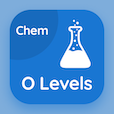Cambridge Online Courses (GCSE)

O Level Chemistry MCQ Questions

O Level Chemistry MCQs PDF - Chapters

# Chemical Formulae and Equations Quiz Answers Online p. 3

Study Chemical Formulae and Equations quiz questions and answers, Chemical Formulae and Equations worksheet with answers key PDF to learn O Level Chemistry MCQ Test 3 for online certification. Chemical Symbols MCQs, Chemical Formulae and Equations MCQ questions and answers for placement and to prepare for job interview. "Chemical Formulae and Equations Quiz" PDF Book: chemical symbols, valency and chemical formula, reactants, relative molecular mass career test for GRE subject tests.

"Variable valencies are used to indicate ions" Multiple Choice Questions (MCQ) on chemical formulae and equations with choices greater than −1, greater than +1, with more than one valency, and none of above for ACT subject tests. Solve chemical symbols quiz questions for jobs' assessment test and online courses for online bachelor degree programs.

## Chemical Formulae & Equations Questions and Answers

MCQ: Variable valencies are used to indicate ions

greater than +1
greater than −1
with more than one valency
none of above

MCQ: In CuO (Copper Oxide), the valency of Copper is

+1
+2
−1
−2

MCQ: In order to get Sodium Sulfite (Na2SO3) as product, the reactants shall be Sulfur Dioxide (SO2) and

Na2CO3
NaOH
NaCl
Na2CO3 and NaOH

MCQ: Relative molecular mass of Na2CO3 is

98
128
286
198

MCQ: Formula of Ammonium Sulfate ((NH4)2SO4) is

NH4(SO4)2
NH4(HSO4)2
(NH4)2SO4
(NH3)2SO3